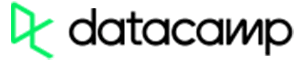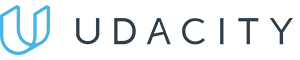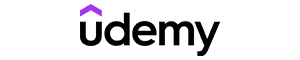Code has been added to clipboard!

# Use PHP Math Functions: Understand Mathematical Processes in Your Code

Published Aug 8, 2017
Updated Oct 2, 2019

Out of eight PHP data types, only two hold numerical values. You can perform the most basic arithmetic actions using operators, such as PHP modulo or PHP division.

For more complex mathematical operations, PHP math functions are used. To start using them, you do not need to install any extensions or packages.

## PHP Math Functions: Main Tips

• PHP math functions are used for handling float and integer variables.
• These functions are inbuilt into PHP.

## List of Functions

In the table below, all the PHP math functions are listed alphabetically. If you are using PHP 4 or a newer version, you can use any of them freely. In case you forget any of these functions, return to this table to refresh your memory! Have a look:

Function Description
abs() Return absolute (positive) value of number
acos() Return arc cosine of number
acosh() Return inverse hyperbolic cosine of number
asin() Return arc sine o number
asinh() Return inverse hyperbolic sine of number
atan() Return arc tangent of number in radians
atan2() Return arc tangent of two variables x, y
atanh() Return inverse hyperbolic tangent of number
base_convert() Convert number from one number base to another
bindec() Convert binary number to decimal number
ceil() Round number up to nearest integer
cos() Return cosine of number
cosh() Return hyperbolic cosine of number
decbin() Convert decimal number to binary number
dechex() Convert decimal number to hexadecimal number
decoct() Convert decimal number to octal number
exp() Calculate exponent of e
expm1() Return exp(x) - 1
floor() Round number down to nearest integer
fmod() Return remainder of x/y
getrandmax() Return largest possible value returned by rand()
hexdec() Convert hexadecimal number to decimal number
hypot() Calculate hypotenuse of right-angle triangle
is_finite() Check if value is finite or not
is_infinite() Check if value is infinite or not
is_nan() Check if value is 'not-a-number'
lcg_value() Return pseudo random number in range between 0 and 1
log() Return natural logarithm of number
log10() Return base-10 logarithm of number
log1p() Return log(1+number)
max() Return highest value in array, or highest value of multiple specified values
min() Return lowest value in array, or lowest value of multiple specified values
mt_getrandmax() Return largest possible value returned by mt_rand()
mt_rand() Generate random integer using Mersenne Twister algorithm
mt_srand() Seed Mersenne Twister random number generator
octdec() Convert octal number to decimal number
pi() Return value of PI
pow() Return x raised to power of y
rand() Generate random integer
round() Round float
sin() Return sine of number
sinh() Return hyperbolic sine of number
sqrt() Return square root of number
srand() Seed random number generator
tan() Return tangent of number
tanh() Return hyperbolic tangent of numberPros
• Easy to use with a learn-by-doing approach
• Offers quality content
• Gamified in-browser coding experience
• The price matches the quality
• Suitable for learners ranging from beginner to advanced
Main Features
• Free certificates of completion
• Focused on data science skills
• Flexible learning timetablePros
• Simplistic design (no unnecessary information)
• High-quality courses (even the free ones)
• Variety of features
Main Features
• Nanodegree programs
• Suitable for enterprises
• Paid Certificates of completionPros
• Easy to navigate
• No technical issues
• Seems to care about its users
Main Features
• Huge variety of courses
• 30-day refund policy
• Free certificates of completion

## Predefined Constants

Now, let's see some predefined constants that are also used in PHP mathematics. The column on the right indicates version of PHP in which a particular constant has been introduced:

Constant Value Description PHP version
INF INF Infinity PHP 4 and newer
M_E 2.7182818284590452354 Return e PHP 4 and newer
M_EULER 0.57721566490153286061 Return Euler constant PHP 4 and newer
M_LNPI 1.14472988584940017414 Return natural logarithm of number PI: log_e(pi) PHP 5.2 and newer
M_LN2 0.69314718055994530942 Return natural logarithm of 2: log_e 2 PHP 4 and newer
M_LN10 2.30258509299404568402 Return natural logarithm of 10: log_e 10 PHP 4 and newer
M_LOG2E 1.4426950408889634074 Return base-2 logarithm of E: log_2 e PHP 4 and newer
M_LOG10E 0.43429448190325182765 Return base-10 logarithm of E: log_10 e PHP 4 and newer
M_PI 3.14159265358979323846 Return Pi PHP 4 and newer
M_PI_2 1.57079632679489661923 Return Pi/2 PHP 4 and newer
M_PI_4 0.78539816339744830962 Return Pi/4 PHP 4 and newer
M_1_PI 0.31830988618379067154 Return 1/Pi PHP 4 and newer
M_2_PI 0.63661977236758134308 Return 2/Pi PHP 4 and newer
M_SQRTPI 1.77245385090551602729 Return square root of number PI: sqrt(pi) PHP 5.2 and newer
M_2_SQRTPI 1.12837916709551257390 Return 2/square root of number PI: 2/sqrt(pi) PHP 4 and newer
M_SQRT1_2 0.70710678118654752440 Return square root of 1/2: 1/sqrt(2) PHP 4 and newer
M_SQRT2 1.41421356237309504880 Return square root of 2: sqrt(2) PHP 4 and newer
M_SQRT3 1.73205080756887729352 Return square root of 3: sqrt(3) PHP 5.2 and newer
NAN NAN Not A Number PHP 4 and newer
PHP_ROUND_HALF_UP 1 Rounds halves up PHP 5.3 and newer
PHP_ROUND_HALF_DOWN 2 Rounds halves down PHP 5.3 and newer
PHP_ROUND_HALF_EVEN 3 Rounds halves to even numbers PHP 5.3 and newer
PHP_ROUND_HALF_ODD 4 Rounds halves to odd numbers PHP 5.3 and newer

## PHP Math Functions: Summary

• PHP math functions don't need to be installed separately, as they are inbuilt.
• They are meant to only be used with variables that contain numerical values: integers and floats.

Days
Hours
Minutes
Seconds